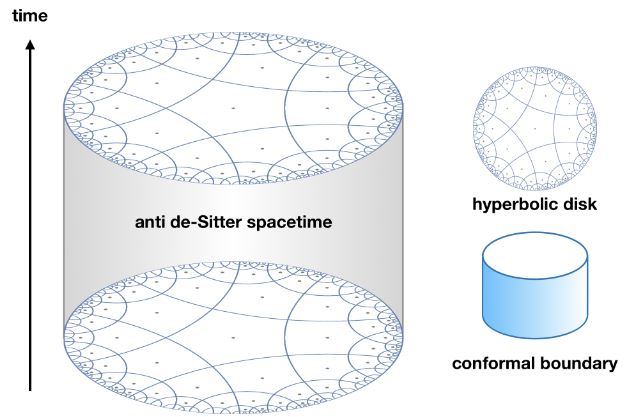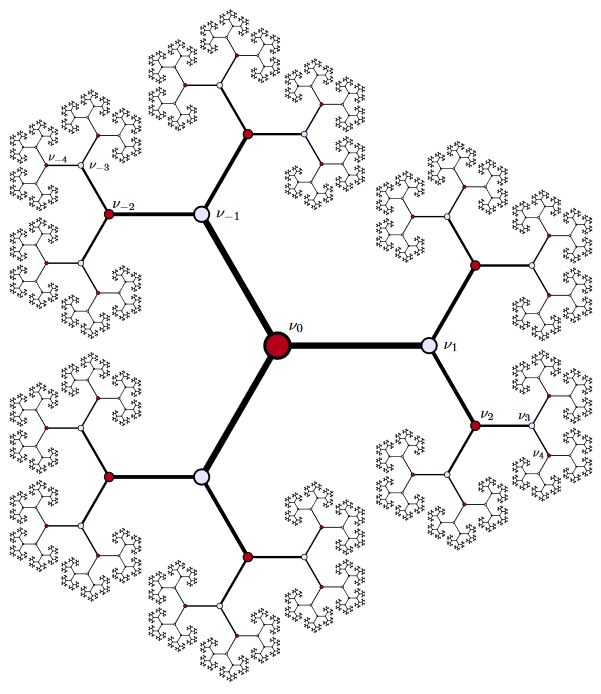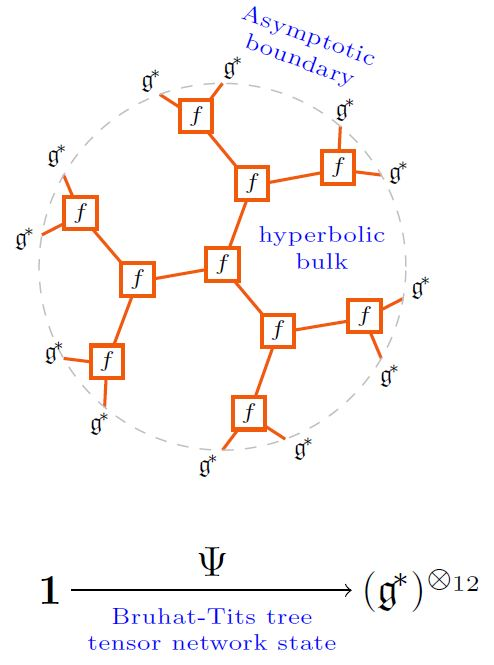# nLab anti de Sitter spacetime

Contents

### Context

#### Riemannian geometry

Riemannian geometry

## Applications

#### Gravity

gravity, supergravity

# Contents## Definition

Up to isometry, the anti de Sitter spacetime of dimension $d$, $AdS_d$, is the pseudo-Riemannian manifold whose underlying manifold is the submanifold of the Minkowski spacetime $\mathbb{R}^{d-1,2}$ that solves the equation

$\sum_{i = 1}^{d-1} (x_i)^2 - (x_d)^ 2 - (x_0)^2 = -R^2$

for some $R \neq 0$ (the “radius” of the spacetime) and equipped with the metric induced from the ambient metric, where $\{x^0, x^1, x^2, \cdots, x^d\}$ denote the canonical coordinates. $AdS_d$ is homeomorphic to $\mathbb{R}^{d-1} \times S^1$, and its isometry group is $O(d-1, 2)$.

More generally, one may define the anti de Sitter space of signature $(p,q)$ as isometrically embedded in the space $\mathbb{R}^{p,q+1}$ with coordinates $(x_1, ..., x_p, t_1, \ldots, t_{q+1})$ as the sphere $\sum_{i=1}^p x_i^2 - \sum_{j=1}^{q+1} t_j^2 = -R^2$.

graphics grabbed from Yan 19

## Properties

### Coordinate charts

(…)

in horospheric coordinates the AdS metric tensor is

$g_{AdS} \;=\; \frac{1}{z^2} \left( g_{(\mathbb{R}^{p,1})} + (d z)^2 \right)$

In terms of

$y \coloneqq 1/z$

this becomes

$g_{AdS} \;=\; y^2 \, g_{(\mathbb{R}^{p,1})} + \frac{1}{y^2}(d y)^2$

and with

$y \coloneqq \tfrac{1}{n} r^n$

for $n \neq 0$

we get

$g_{AdS} \;=\; \tfrac{1}{n^2}r^{2n} \, g_{(\mathbb{R}^{p,1})} + \frac{1}{r^2}(d r)^2$

(…)

### Holography

Asymptotically anti-de Sitter spaces play a central role in the realization of the holographic principle by AdS/CFT correspondence.

### In $p$-adic geometry

A 2-adic arithmetic geometry-version of AdS spacetime is identified with the Bruhat-Tits tree for the projective general linear group $PGL(2,\mathbb{Q}_p)$:graphics from Casselman 14

In the p-adic AdS/CFT correspondence this may be regarded (at some finite depth truncation) as a tensor network state:graphics from Sati-Schreiber 19c

and as such validates the Ryu-Takayanagi formula for holographic entanglement entropy.

### General

Reviews:

Further discussion:

• Abdelghani Zeghib, On closed anti de Sitter spacetimes, Math. Ann. 310, 695–716 (1998) (pdf)

• C. Frances, The conformal boundary of anti-de Sitter space-times, in AdS/CFT correspondence: Einstein metrics and their conformal boundaries , 205–216, IRMA Lect. Math. Theor. Phys., 8, Eur. Math. Soc., Zürich, 2005 (pdf)

• Jiri Podolsky, Ondrej Hruska, Yet another family of diagonal metrics for de Sitter and anti-de Sitter spacetimes, Phys. Rev. D 95, 124052 (2017) (arXiv:1703.01367)

Discussion of thermal Wick rotation on global anti-de Sitter spacetime (which is already periodic in real time) to Euclidean field theory with periodic imaginary time is in

Discussion of black holes in anti de Sitter spacetime:

• Hawking, Stephen W., and Don N. Page. “Thermodynamics of black holes in anti-de Sitter space.” Communications in Mathematical Physics 87.4 (1983): 577-588.

• M. Socolovsky, Schwarzschild Black Hole in Anti-De Sitter Space (arXiv:1711.02744)

• Peng Zhao, Black Holes in Anti-de Sitter Spacetime (pdf)

• Jakob Gath, The role of black holes in the AdS/CFT correspondence (pdf)

Relation to Teichmüller theory:

• Francesco Bonsante, Andrea Seppi, Anti-de Sitter geometry and Teichmüller theory (arXiv:2004.14414)

### As string vacua

On (in-)stability of non-supersymmetric AdS vacua in string theory:

### pp-Waves as Penrose limits of $AdS_p \times S^q$ spacetimes

Discussion of pp-wave spacetimes as Penrose limits (Inönü-Wigner contractions) of AdSp x S^q spacetimes and of the corresponding limit of AdS-CFT duality:

Review:

• Michael Gutperle, Nicholas Klein, A Penrose limit for type IIB $AdS_6$ solutions (arXiv:2105.10824)